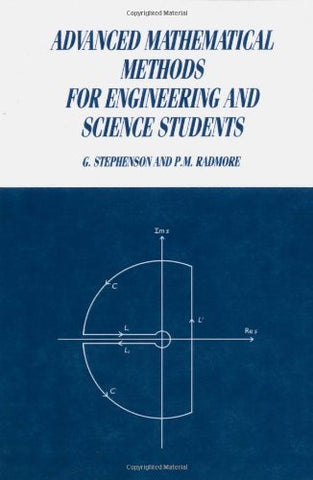# Advanced Mathematical Methods for Engineering and Science Students

• \$76.94
• Save \$29
• Binding: Paperback
• Author: G. Stephenson;P. M. Radmore
• Publish Date: 1990-04-27

Attention : For textbook, access codes and supplements are not guaranteed with used items.

This textbook provides a solid foundation to a number of important topics in mathematics of interest to science and engineering students. Included are tensor algebra, ordinary differential equations, contour integration, Laplace and Fourier transforms, partial differential equations and the calculus of variations. The authors' approach is simple and direct with an emphasis on the analytical understanding of the material. The text is virtually selfcontained, assuming only that the student has a solid understanding of ancillary mathematics. Each chapter contains a large number of worked examples, and concludes with problems for solution, with answers in the back of the book.